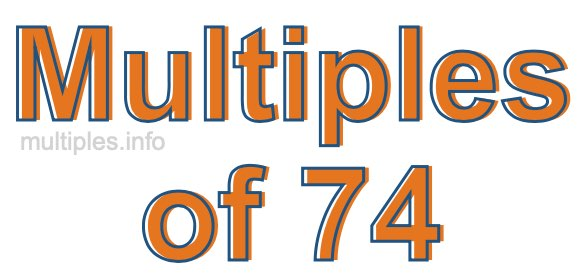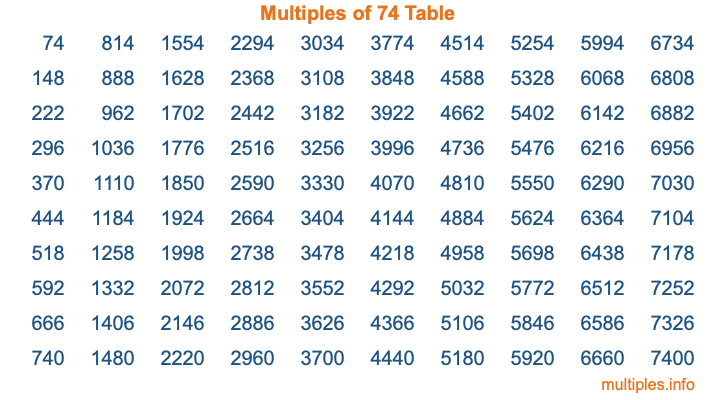Multiples of 74Welcome to the Multiples of 74 page. Here we will first teach you everything you will ever need to know about the multiples of 74, and then give you a study guide summary of everything we taught you to make sure you remember it all. Use this page to look up facts and learn information about the multiples of 74. This page will make you a multiples of seventy-four expert!

Definition of Multiples of 74
Multiples of 74 are all the numbers that when divided by 74 equal an integer. Each of the multiples of 74 are called a multiple. A multiple of 74 is created by multiplying 74 by an integer.

Therefore, to create a list of multiples of 74, you start with 1 multiplied by 74, then 2 multiplied by 74, then 3 multiplied by 74, and so on for as long as you want. Thus, the list of the first five multiples of 74 is 74, 148, 222, 296, and 370. To see a larger list of multiples of 74, see the printable image of Multiples of 74 further down on this page. We also have a category where you can choose any nth multiple of 74.

Multiples of 74 Checker
The Multiples of 74 Checker below checks to see if any number of your choice is a multiple of 74. In other words, it checks to see if there is any number (integer) that when multiplied by 74 will equal your number. To do that, we divide your number by 74. If the the quotient is an integer, then your number is a multiple of 74.

Is  a multiple of 74?

Least Common Multiple of 74 and ...
A Least Common Multiple (LCM) is the lowest multiple that two or more numbers have in common. This is also called the smallest common multiple or lowest common multiple and is useful to know when you are adding our subtracting fractions. Enter one or more numbers below (74 is already entered) to find the LCM.

Check out our LCM Calculator if you need more details about the Least Common Multiple or if you need the LCM for different numbers for adding and subtraction fractions.

nth Multiple of 74
As we stated above, 74 is the first multiple of 74, 148 is the second multiple of 74, 222 is the third multiple of 74, and so on. Enter a number below to find the nth multiple of 74.

th multiple of 74

Multiples of 74 vs Factors of 74
74 is a multiple of 74 and a factor of 74, but that is where the similarities end. All postive multiples of 74 are 74 or greater than 74. All positive factors of 74 are 74 or less than 74.

Below is the beginning list of multiples of 74 and the factors of 74 so you can compare:

Multiples of 74: 74, 148, 222, 296, 370, etc.

Factors of 74: 1, 2, 37, 74

As you can see, the multiples of 74 are all the numbers that you can divide by 74 to get a whole number. The factors of 74, on the other hand, are all the whole numbers that you can multiply by another whole number to get 74.

It's also interesting to note that if a number (x) is a factor of 74, then 74 will also be a multiple of that number (x).

Multiples of 74 vs Divisors of 74
The divisors of 74 are all the integers that 74 can be divided by evenly. Below is a list of the divisors of 74.

Divisors of 74: 1, 2, 37, 74

The interesting thing to note here is that if you take any multiple of 74 and divide it by a divisor of 74, you will see that the quotient is an integer.

Multiples of 74 Table
Below is an image of the first 100 multiples of 74 in a table. The table is in chronological order, column by column. The first column has the first ten multiples of 74, the second column has the next ten multiples of 74, and so on.The Multiples of 74 Table is also referred to as the 74 Times Table or Times Table of 74. You are welcome to print out our table for your studies.

Negative Multiples of 74
Although not often discussed or needed in math, it is worth mentioning that you can make a list of negative multiples of 74 by multiplying 74 by -1, then by -2, then by -3, and so on, to get the following list of negative multiples of 74:

-74, -148, -222, -296, -370, etc.

Multiples of 74 Summary
Below is a summary of important Multiples of 74 facts that we have discussed on this page. To retain the knowledge on this page, we recommend that you read through the summary and explain to yourself or a study partner why they hold true.

There are an infinite number of multiples of 74.

A multiple of 74 divided by 74 will equal a whole number.

74 divided by a factor of 74 equals a divisor of 74.

The nth multiple of 74 is n times 74.

The largest factor of 74 is equal to the first positive multiple of 74.

74 is a multiple of every factor of 74.

74 is a multiple of 74.

A multiple of 74 divided by a divisor of 74 equals an integer.

74 divided by a divisor of 74 equals a factor of 74.

Any integer times 74 will equal a multiple of 74.

Multiples of a Number
Here you can get the multiples of another number, all with the same attention to detail as we did for multiples of 74 on this page.

Multiples of
Multiples of 75
Did you find our page about multiples of seventy-four educational? Do you want more knowledge? Check out the multiples of the next number on our list!